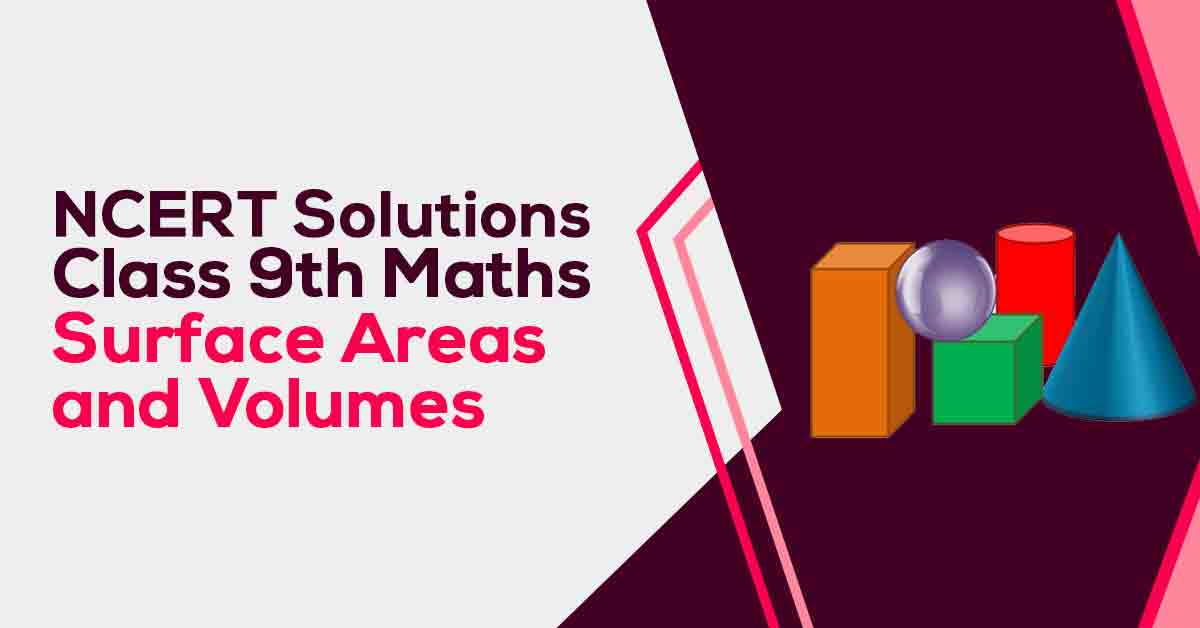NCERT Solutions for Class 9 Maths Chapter 13 | Vidyakul
×

# NCERT Solutions For Class 9 Maths Chapter 13

## NCERT Solutions For Class 9 Maths Chapter 13 Surface Areas and Volumes - PDF DownloadStudents require NCERT Solutions for Class 9 Maths Chapter 13 Surface Areas and Volumes for better exam preparation. In this chapter, we will learn that the Surface area of a cuboid = 2 (lb + bh + hl). The surface area of a cube = 6a2. Curved surface area of a cylinder = 2πrh. Total surface area of a cylinder = 2πr(r + h).

These CBSE NCERT Solutions for Class 9 Maths Chapter 13 Surface Areas and Volumes will help you to practice all the topics in the chapter with ease and score maximum marks in the examination.